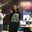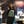Trusted answers to developer questions
Trusted Answers to Developer Questions

Related Tags

theoretical cs
automata

# What is Chomsky's hierarchy?Ayesha Kanwal

Grokking Modern System Design Interview for Engineers & Managers

Ace your System Design Interview and take your career to the next level. Learn to handle the design of applications like Netflix, Quora, Facebook, Uber, and many more in a 45-min interview. Learn the RESHADED framework for architecting web-scale applications by determining requirements, constraints, and assumptions before diving into a step-by-step design process.

### Chomsky's hierarchy

According to Chomsky's hierarchy, four basic types of grammar are used in the automaton theory. These are the following:

• Type 0
• Type 1
• Type 2
• Type 3
Scope of grammars

### Types of grammar

The four types of grammar are explained below.

#### Type 0

The productions in this type 0 have no restrictions. This type of grammar includes all types of formal grammar.

The productions produced by this grammar are of the format $\alpha \rightarrow \beta$. In this grammar, $\alpha$ is generally a string of terminals and nonterminals, along with at least one nonterminal. $\alpha$ can not be null. $\beta$ is also a string of terminals and nonterminals.

• Accepted grammar: The accepted grammar for type 0 is unrestricted.
• Language accepted: The accepted language for type 0 is recursively enumerable.
• Automaton: The automaton that accepts type 0 is a Turing machine.
##### Example

The following is an example of type 0 grammar:

#### Type 1

Type 1 grammar produces productions in the format $\alpha A \beta \rightarrow \alpha \gamma \beta$. In this, $\alpha$, $\beta$, and $\gamma$ are strings of terminals and nonterminals. $A$ is a nonterminal.

There is an exception in this grammar. It is that the rule $S\rightarrow\epsilon$ is not to be produced if $S$ occurs on the right side of the rule in the whole grammar.

• Accepted grammar: The accepted grammar for type 1 is context-sensitive grammar.
• Language accepted: The accepted language for type 1 is context-sensitive language.
• Automaton: The automaton that accepts type 1 is linearly bound.
##### Example

The following is an example of type 1 grammar:

#### Type 2

Type 2 grammar produces rules in the form of $A \rightarrow \alpha$. In this form, $\alpha$ is a string of terminals and nonterminals, and $A$ is a nonterminal.

• Accepted grammar: The accepted grammar for type 2 is context-free grammar.
• Language accepted: The accepted language for type 2 is context-free language.
• Automaton: The automaton that accepts type 2 is a pushdown automaton.
##### Example

The following is an example of type 2 grammar:

#### Type 3

Type 3 grammar has a nonterminal on the left side of the arrow and a nonterminal or a combination of a terminal and a nonterminal.

The productions produced are in the form of $S\rightarrow a$ or $S \rightarrow aT$. Where $a$ is a terminal and $S$ and $T$ are nonterminals.

The production $S\rightarrow \epsilon$ can be produced if $S$ does not occur on the right side of any rule.

• Accepted grammar: The accepted grammar for type 3 is regular.
• Language accepted: The accepted language for type 3 is regular.
• Automaton: The accepted automaton for type 3 is a finite state automaton.
##### Example

The following is an example of type 3 grammar:

RELATED TAGS

theoretical cs
automata

CONTRIBUTORAyesha Kanwal